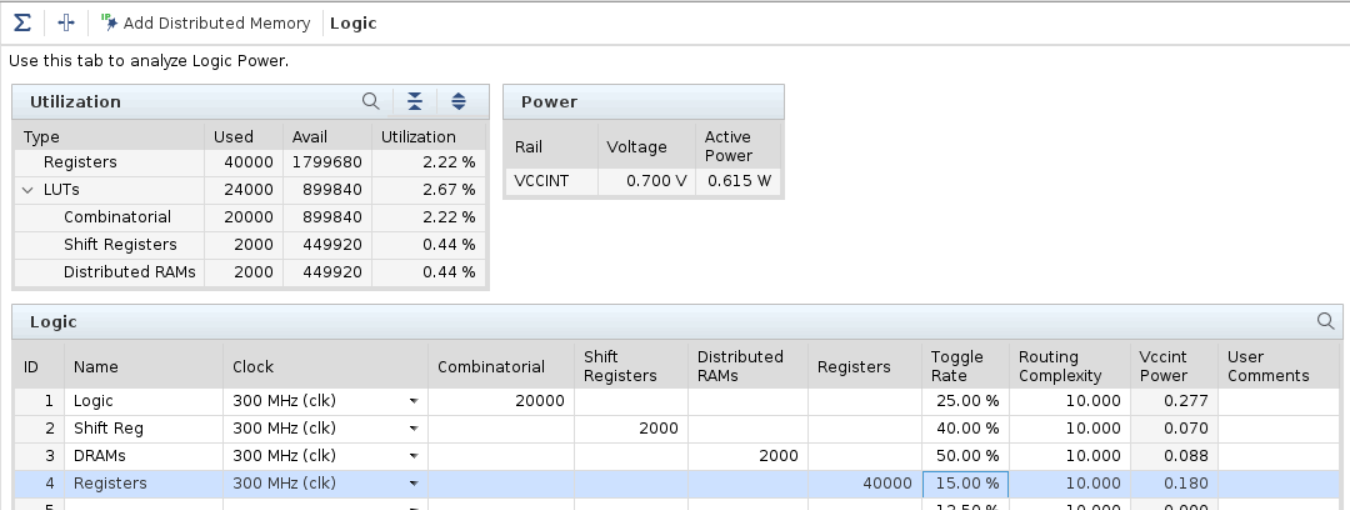# Logic - 2022.2 English

## Power Design Manager User Guide (UG1556)

Document ID
UG1556
Release Date
2022-10-19
Version
2022.2 English

The Logic sheet covers power estimates of CLB logic: LUTs and Registers as shown in the following figure. Each row represents a group of logic that is associated with:

• A particular Clock whose frequency is used to calculate dynamic power.
• A Toggle Rate that represents an average over the inputs and outputs of all logic.
LUTs fall into the following three categories, while registers are primarily the CLB registers.
LUT as Combinatorial logic
For simplified entry, PDM assumes an average sized LUT of about five inputs and also assumes a small percentage of LUTs use two outputs.
LUT as Shift Registers
SRL primitives
LUT as Distributed RAMs
LUTRAM primitives
Figure 1. PDM LogicBoth Shift Registers and Distributed RAMs use the M-type CLB LUTs that you can configure as memory. To eliminate the difficulties of estimating total LUTs used for distributed RAM-based memories, use the Add Memory button to launch the PDM Memory Configuration wizard. Specify the memory array size, clock and options, and the PDM tool calculates the expected number of LUTs and registers and enters them into a row. Toggle Rate is defined as the percentage of clock cycles where a transition occurs. The default value of 12.5% means one transition every eight cycles.

Routing Complexity column is an abstract model of the interconnect power. The number represents the average number of routing resources per logical net. A design with higher complexity requires more routing resources per net which increases power. Routing Complexity is typically only modified when importing Vivado power analysis results where Routing Complexity is calculated from the actual routing resources that are used to route the design. For early estimation, Xilinx recommends that you leave Routing Complexity at the default setting.